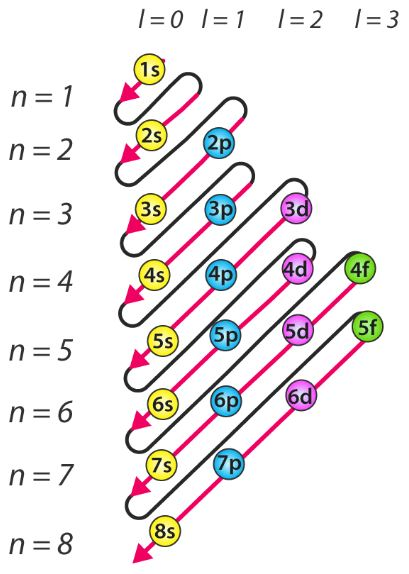Page 1 of 1

### s, p, d, f orbitals

Posted: Tue Oct 22, 2019 10:31 pm
Which has more energy, the 4s orbital or 3d orbital? Are there any exceptions or special rules to keep in mind when comparing the two orbitals ?

### Re: s, p, d, f orbitals

Posted: Tue Oct 22, 2019 10:46 pm
The 4s orbitals have a lower energy than the 3d, and so the 4s orbitals are filled first. When writing electron configurations the order in the orbitals are filled in increasing order of energy levels so 4s would come before 3d.

### Re: s, p, d, f orbitals

Posted: Tue Oct 22, 2019 10:50 pm
As to the first part of your question, 3d orbitals have slightly higher energy than 4s orbitals, thus, the 4s orbitals get filled first. This is only true if there are no electrons in the 3d orbitals. If the 3d orbitals are filled, then the 4s orbitals will be higher in energy.

### Re: s, p, d, f orbitals

Posted: Tue Oct 22, 2019 10:53 pm
The 4s orbital has more energy than the 3d orbital. However, for elements like potassium and calcium, the 3d orbitals have a higher energy than the 4s, as the 4s levels fill before than the 3d.
But for the next 10 elements of the transition series, the 3d orbitals gradually fill with electrons, and so in this case the 3d orbital does not have higher energy than the 4s.

### Re: s, p, d, f orbitals

Posted: Tue Oct 22, 2019 10:53 pm
The 4s orbital has lower energy than the 3d orbital because it is filled up first than the 3d level. when writing it down, its recommended to write it in the format of s,p,d,f. That's what I was taught.

### Re: s, p, d, f orbitals

Posted: Tue Oct 22, 2019 10:57 pm
The s-orbital is filled up first but when the s-orbital is occupied and electrons enter the d-orbital, the d-orbital will have a lower energy than the s-orbital. For example, 3d has a lower energy than 4s when electrons are in the 3d orbital and 4s is occupied. So when writing out the electron configuration, place 3d before 4s (this applies only to elements with a proton number greater than 20).

Ex. for the element Sc: [Ar] 3d^1 4s^2

There are two exceptions for Chromium and Copper where the 4s orbital is not fully occupied but the 3d orbital still has a lower energy.

Cr: [Ar] 3d^5 4s^1 (this occurs so all the d-orbitals are half full)
Cu: [Ar] 3d^10 4s^1 (this occurs so all the d-orbitals are full)

### Re: s, p, d, f orbitals

Posted: Tue Oct 22, 2019 11:01 pm
A question I do have is that when reviewing the notes in Lecture, it states that 4s, 4p has higher energy than 1s, 2s,2p, 3s,3p, and 3d. So I am quite confused and perplexed on how the orbitals work.

### Re: s, p, d, f orbitals

Posted: Tue Oct 22, 2019 11:09 pm
Victoria Otuya 4F wrote:A question I do have is that when reviewing the notes in Lecture, it states that 4s, 4p has higher energy than 1s, 2s,2p, 3s,3p, and 3d. So I am quite confused and perplexed on how the orbitals work.

From what I understand, the 4s and 4p subshells are higher in energy than 1s, 2s,2p, 3s,3p, and 3d. When there are electrons in 4s, it will be higher in energy than 3d. This is why, when writing electron configurations, we have been writing 3d before 4s.

### Re: s, p, d, f orbitals

Posted: Wed Oct 23, 2019 12:44 am
This picture shows the energy levels of all the orbitals:### Re: s, p, d, f orbitals

Posted: Wed Oct 23, 2019 11:18 am
so at each energy level, are there s- p- d- f- orbitals?

### Re: s, p, d, f orbitals

Posted: Thu Oct 24, 2019 3:49 pm
PriscillaLi_3G wrote:so at each energy level, are there s- p- d- f- orbitals?

In the n=1 energy level, there is only the s-orbital. In n=2, there is only the s-orbital and p-orbital. In n=3, there is only the s-, p-, d-orbitals. Another way to tell which orbitals are present at an energy level is by using the quantum numbers. The quantum number l = 0, 1, 2, 3 corresponds with s, p, d, and f respectively. The quantum number l has values l = 0, 1, 2, 3, 4..., (n-1).

### Re: s, p, d, f orbitals

Posted: Thu Oct 24, 2019 9:52 pm
So do we place them in order by that image or by n?

### Re: s, p, d, f orbitals

Posted: Fri Oct 25, 2019 8:46 am
The 3D orbital is slightly higher in energy; visually, if you look at the periodic table, it can be seen that the 4s orbital comes before 3D.

### Re: s, p, d, f orbitals

Posted: Fri Oct 25, 2019 10:02 am
The 4s level has a lower energy level than 3d which is why the 4s subshell gets filled with electrons first and the 3d after. However, when writing the electron configuration you should put 3d before 4s.

### Re: s, p, d, f orbitals

Posted: Fri Oct 25, 2019 11:17 am
http://hyperphysics.phy-astr.gsu.edu/hb ... rfill.html

The first diagram on this page helped me a lot with figuring out the order of e- configurations and their energies.

### Re: s, p, d, f orbitals

Posted: Fri Oct 25, 2019 4:48 pm
The 3d orbital should have more energy than 4s since the electrons in the 3d orbital experience heavy repulsion from electron interactions between the other electrons in the 3d and 3p orbitals. As a result, it requires less energy for electrons to occupy the 4s orbital.